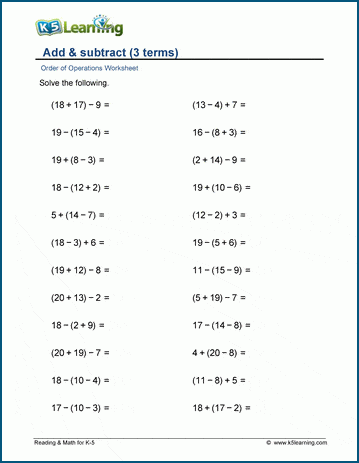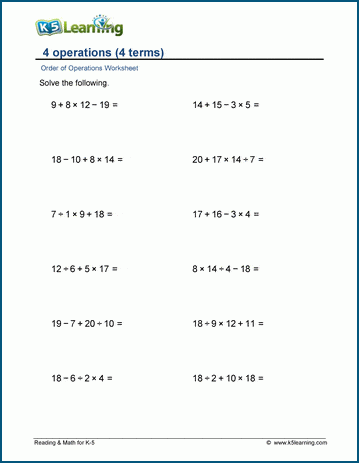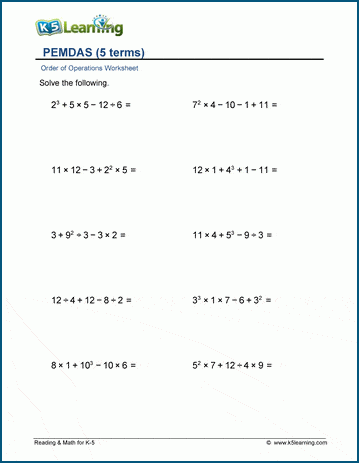# Order of Operations Math Drill Practice

The math drill worksheets in this section cover the order of operations for addition, subtraction, multiplication, and division, as well as worksheets covering exponents.

## Add and subtract with parenthesis worksheets

This area covers adding and subtracting 3-4 terms with parenthesis, and a more difficult set of worksheets with 5 and more terms with parenthesis.

The below worksheet covers addition and subtraction of 3 terms with parenthesis.## Four operations with parenthesis practice

The next section covers addition, subtraction, multiplication, and division with parenthesis. The sets of worksheets vary in degree of difficult in starting with 3 terms, then 4 terms and finally 5 terms, all without and with parenthesis.

The below worksheet covers 4 terms with no parenthesis.## Practice exponents and parentheses with four operations

This final sub-section covers both parentheses and exponents in worksheet with all four operations. Again, they vary in difficulty, starting with 3 terms, then 4 terms and finally 5 terms.

The below worksheet covers 5 terms with exponents and no parenthesis. Further worksheets cover both parentheses and exponents.